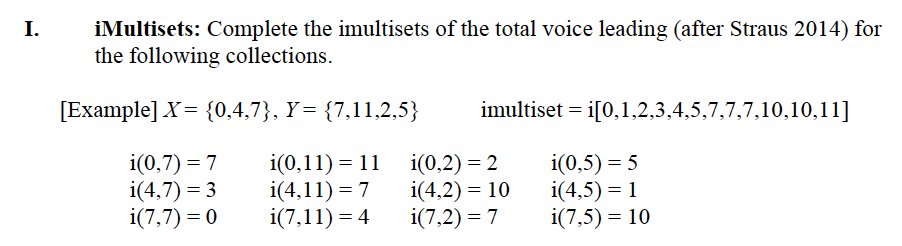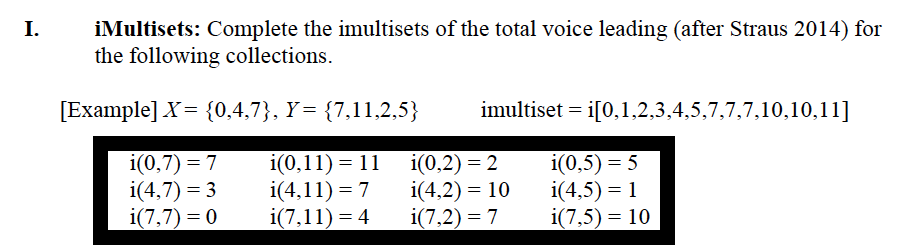# Calculating iMultisets in R

November is pretty much the worst month for people in higher education. There are too many deadlines and if you’re still in coursework (like myself) you have essays to write, presentations to make, and a backlog of homework assignments to grade. So if you can save time here or there, it’s usually a good choice.

This weekend I was working a homework assignment for my Transformational Theory seminar where we were given a number of pairs of pitch class sets and had to calculate the imultiset for each following Joseph Straus’ 2014 article on Total Voice Leading.

As I looked at the top of the assignment (pictured below) and started to crank out the first one by hand, I realized that the next 30 minutes of my life were going to be doing the same thing over and over again.Usually if I get that feeling my next thought is “Can I make a computer do this?” and after thinking about it for two minutes I realized the answer was yes.

So instead of doing all of these by hand, I wrote an R script and with the time saved figured I’d write a quick post about it.

## The Problem

In order to calculate the imultiset you need two pitch class sets, in this case X and Y. Each set can have any number of pitch classes in them and what you need to do is calculate the distance in Modulo 12 space between every possible combination of pitch classes from one set to the other. So for example, you could move from 4 (E in Mod 12 for you non-music theory readers) to 7, 11, 2, or 5 (G, B, D, or F) resulting in four intervals: {3,7,10,1}. These four numbers in the {curly braces} are what you get when you subtract each number in the second set from the note E in Mod 12 space. This action then needs to be completed for every pair.

When you need to account for every pairing you need to do a cross join. A cross join connects each member of one set to each member of another set. This creates the sets of pairs seen below.Then all you need to do is subtract one from the other to get the distance. The only problem is that these subtractions need to happen in Mod 12 space so in any case where you are subtracting a bigger number from a smaller number you will get a negative result! This is easily fixed by just adding 12 to that number in order to get what we should have been our answer if we were doing Mod 12 arithmetic.

After fixing the Mod 12 problem, you’ll have a nice list of intervals that just have to be sorted from top to bottom to have your imultiset. So let’s see how you would do this line by line.

## R Code

First, let’s get two pitch class sets. In this case we have a C major triad and a G7 chord.

X = {0,4,7} and Y = {7,11,2,5}

Let’s first assign each chord to an object.

X <- c(0,4,7)
Y <- c(7,11,2,5)

Next we need to do that cross join, which we can accomplish with R’s merge() function. This makes us a data frame with every combination from set X and set Y. Below we see the function’s output.

Example <- merge(X,Y,all=TRUE)
Example
##    x  y
## 1  0  7
## 2  4  7
## 3  7  7
## 4  0 11
## 5  4 11
## 6  7 11
## 7  0  2
## 8  4  2
## 9  7  2
## 10 0  5
## 11 4  5
## 12 7  5

Once having each combination, we then subtract one set from the other. Since I don’t know how to put R into Music Theory Mode where it only operates in Mod 12, we can fix the problem of the negative numbers by just indexing through our answer with in ifelse() statement to replace any negative values with the answer we actually want by adding 12 to it.

Example$diff <- Example$y - Example$x Example$diff
##    7  3  0 11  7  4  2 -2 -5  5  1 -2
Example$mod12 <- ifelse(test = Example$diff < 0 ,
yes = Example$diff + 12, no = Example$diff)
Example$mod12 ##  7 3 0 11 7 4 2 10 7 5 1 10 With our numbers then in Mod 12 space, we just sort them and we get our imultiset. sort(Example$mod12)
##    0  1  2  3  4  5  7  7  7 10 10 11

Of course you are not going to want to write this out every time you want to calculate an imultiset, so best to just write a function that does what we just did.

calculate.multiset <- function(x,y){
array.1 <- x
array.2 <- y
cross.join <- merge(array.1,array.2, all = TRUE)
cross.join$diff <- cross.join$y - cross.join$x cross.join$mod12 <- ifelse(cross.join$diff < 0, cross.join$diff + 12, cross.join$diff) sort(cross.join$mod12)
}